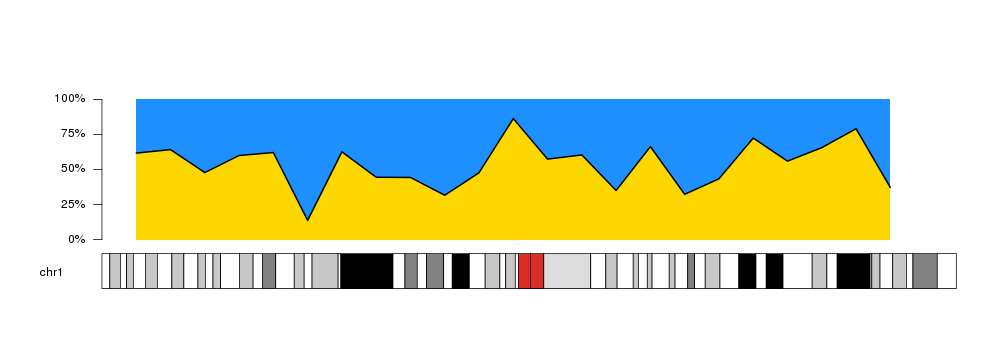Plotting Areas

kpArea is similar to kpLines but in addition to plotting the defined line, it fills the area below the line with the specified color. It might be a useful representation for coverage, or for continuous values such as methylation levels, etc.

library(karyoploteR)

x <- 1:23*10e6
y <- rnorm(23, mean=0.5, sd=0.2)

kp <- plotKaryotype(chromosomes="chr1")
kpArea(kp, chr="chr1", x=x, y=y)
kpAxis(kp, ymin = 0, ymax=1)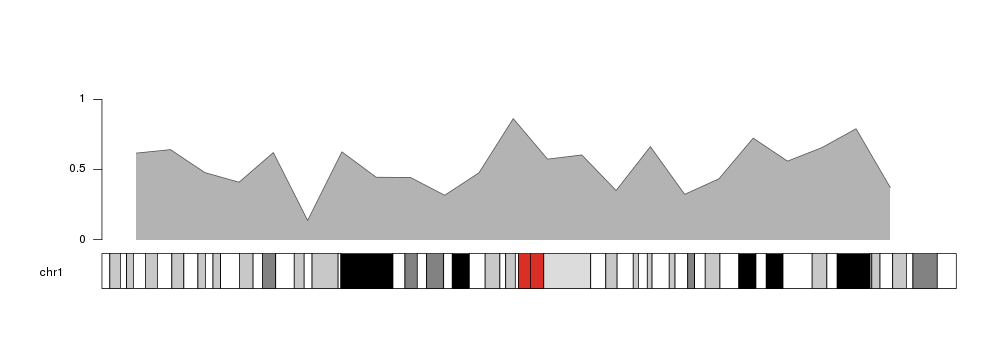kpAreahas an additional parameter, base.y=0, that specifies the y level at which the area ends. Its default value is 0, so the area will be shaded between the outer line and 0. That means that if part of the line is below 0, the shading will be above the outer line.

y <- -0.8

kp <- plotKaryotype(chromosomes="chr1")
kpArea(kp, chr="chr1", x=x, y=y, ymin=-1, ymax=1)
kpAxis(kp, ymin = -1, ymax=1)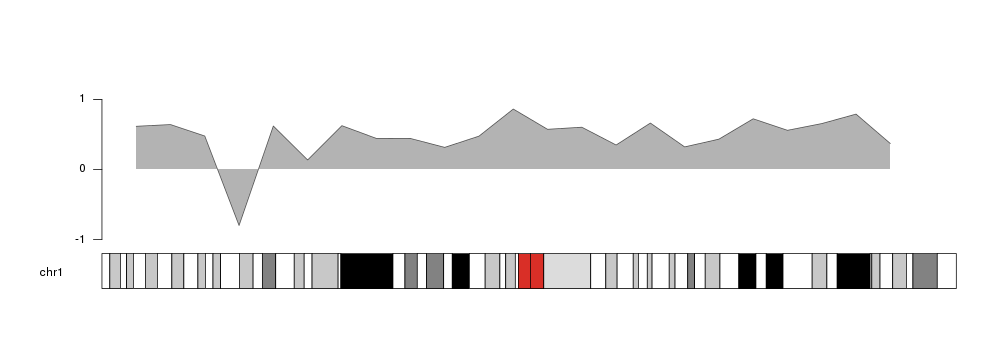Following the same principle, we can change the value of base.y to shade the area above the outer line.

y <- 0.6

kp <- plotKaryotype(chromosomes="chr1")
kpArea(kp, chr="chr1", x=x, y=y, base.y=1)
kpAxis(kp, ymin=0, ymax=1)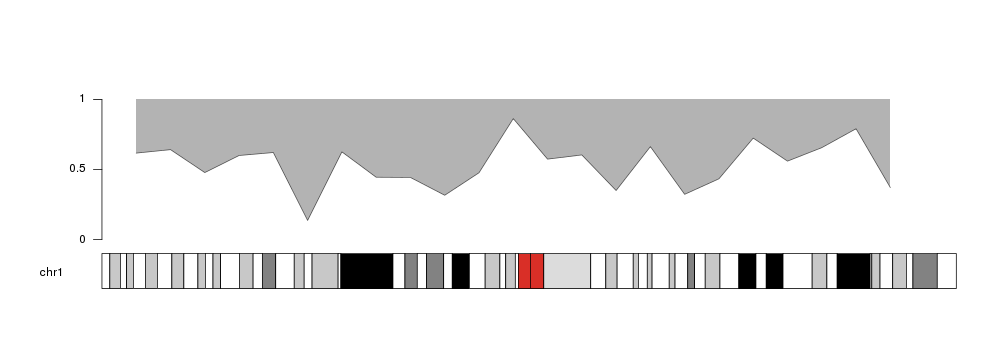It is possible to specify different colors for the outer line and the shaded area, as well as other standard graphical parameters used in the R base graphics: lwd, lty… In addition, density and angle can be used to fill the area with shading lines as in the polygon function.

For colors, col specifies the color of the shaded area. If no color is given (col=NULL) it will be filled with a lighter version of the line color. Similarly, border defines the color of the outer line and it will take a darker shade of of the area color if border=NULL. If any of these parameters is NA the element (area or line) will not be drawn.

kp <- plotKaryotype(chromosomes="chr1")
kpArea(kp, chr="chr1", x=x, y=y, col="gold", r0=0, r1=0.2)
kpArea(kp, chr="chr1", x=x, y=y, border="gold", r0=0.2, r1=0.4)
kpArea(kp, chr="chr1", x=x, y=y, col="gold", border="red", density=20, r0=0.4, r1=0.6)
kpArea(kp, chr="chr1", x=x, y=y, col="gold", border=NA, r0=0.6, r1=0.8)
kpArea(kp, chr="chr1", x=x, y=y, col="gold", lty=2, lwd=3, r0=0.8, r1=1)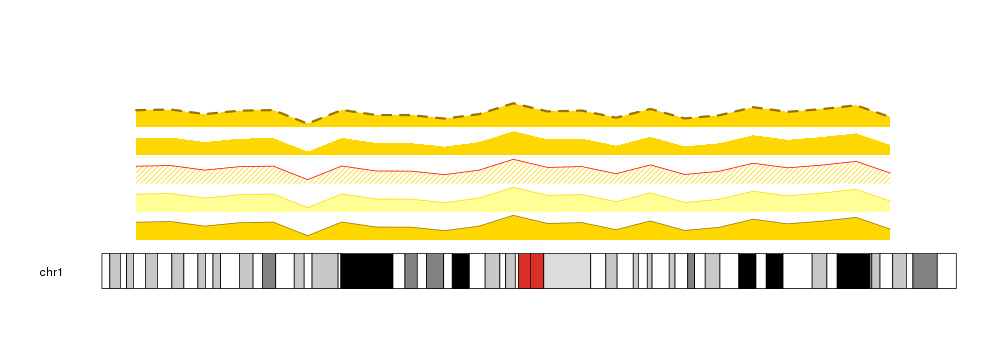Combining this options we can create other variants of the plot, and for example use it to represent proportions over the genome.

kp <- plotKaryotype(chromosomes="chr1")
kpArea(kp, chr="chr1", x=x, y=y, border=NA, col="#FFD700")
kpArea(kp, chr="chr1", x=x, y=y, col="#1E90FF", border="black", lwd=2, base.y=1)
kpAxis(kp, ymin=0, ymax=100, numticks = 5, labels = c("0%", "25%", "50%", "75%", "100%"))